# Algebra II : Variable Relationships

## Example Questions

2 Next →

### Example Question #21 : Graphing Data

Given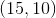and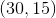use linear interpolation to findwhen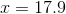.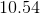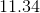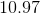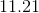Explanation:

Use the formula for interpolation: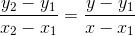We will use (30, 15) as x2 and y2, (15, 10) as x1 and y1, and solve for y when x=17.9: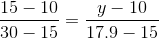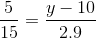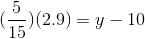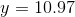### Example Question #22 : Graphing Data

Mary measures her height every year on her birthday, starting at 11 until she turns 16. She wants to make a table with all the information gathered, but discovers she lost the piece of paper on which she wrote her height down on her 14th birthday. Her incomplete table looks like this:

 Age (years) Height (inches) 11 47.5 12 50.25 13 53 14 ? 15 58.5 16 61.25

Using the method of linear interpolation, which of the following is the closest estimate of Mary's height on her 14th birthday?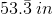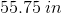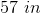Not enough information given in the problem.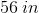Explanation:

Using linear interpolation means that we draw a line between the points on our data set and use that line to estimate a value that lies between two data points; in this case, we have the data from Mary's 13th and 15th birthdays, so we can describe a line between those two points and estimate her height at 14. Our line will be written in slope-intercept form:Where the variablerepresents Mary's Age in years and the variablerepresents her height in inches. First, we need to find the slope. Using 2 points on our table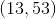and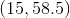as point 1 and point 2, respectively, we plug these values into our slope formula: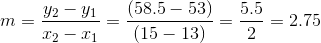Next, we find the y-intercept by plugging in our slope (which we just found) and a point from our table (we'll stick with) and solving for: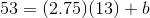Simplify: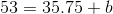Subtract 35.75 from both sides to solve for: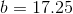The equation of our interpolation line is: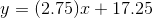So, to get an estimate of Mary's height on her 14th birthday, we plug in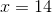and solve for: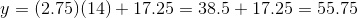Our estimate of Mary's height atis### Example Question #441 : Basic Statistics

Find the value ofwhen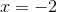given the points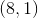and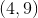.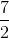Explanation:

Write the interpolation formula.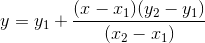Identify and substitute the values.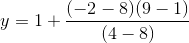Simplify the fraction.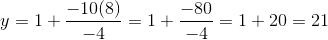The answer is:### Example Question #1 : Extrapolations

What is the next number in this sequence: 8, 27, 64, 125 ?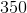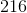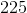Explanation:

Find the pattern of the sequence:

This pattern is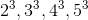so the next number in the sequence would be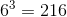### Example Question #1 : Extrapolations

Find the next 2 numbers in this sequence: 33, 46, 72, 111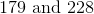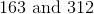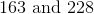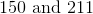Explanation:

Find the pattern in this sequence of numbers:

In this case, the pattern is adding 13n to the previous number where n= how many numbers came before the current number.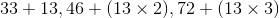so the first number we are looking for would be: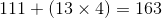the second number we are looking for would be: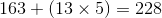### Example Question #3 : Extrapolations

The amount of water inside of a leaky boat is measured periodically after the boat has been in the water in different periods of time and are found to have a linear relationship. The results are given in the following chart:

 Time in water (mins) Amount of water in the boat (gal) 0 0 6 4.8 19 15.2 28 22.4

Using the method of linear extrapolation based on the data from the table, how much water would you expect to be in the boat after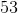minutes?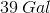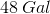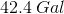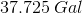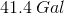Explanation:

To extrapolate the results of the study out to 53 minutes, first we have to determine an equation representing the relationship between time passed and amount of water; we can write our equation in slope-intercept form:Where our y-axis represents amount of water and the x-axis represents time. We can pick 2 points and label them Point 1 and Point 2; looking back at the table:

 Time in water (mins) Amount of water in the boat (gal) 0 0 6 4.8 19 15.2 28 22.4

We label Point 1 as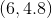and Point 2 as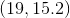; we plug these points into the slope formula as follows: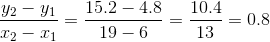So, the slope of our line that describes how much water is in the boat is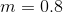; to find ourterm, the y-intercept, we need to pick a point on the graph and plug in our slope to solve for y-intercept. Let's once again choose the point: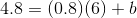Simplify the expression and we find that b=0, so our slope-intercept equation is: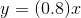Plugging in a value of 53 for, we find that: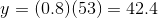So the answer is 42.4 gallons of water in the boat after 53 minutes.

2 Next →

### All Algebra II Resources